Question

# 24. Which structure for dinitrogen sulfide (N-N S or NSN) is the more stable? a. N...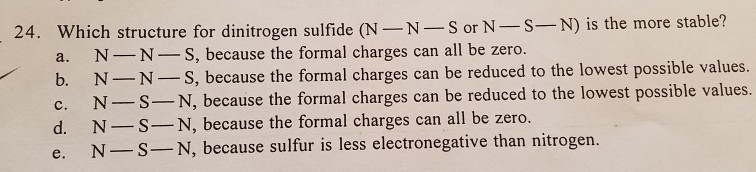24. Which structure for dinitrogen sulfide (N-N S or NSN) is the more stable? a. N N S, because the formal charges can all be zero. b. N-NS, because the formal charges can be reduced to the lowest possible values I charges can be reduced to the lowest possible values d. N-S-N, because the formal charges can all be zero. s electronegative than nitrogen.

24)

Formal charge = ( Number of valence electrons on atom) -(non-bonding valence electrons+ bonding electrons/2)

For N-N-S

Formal charge: 5-(2+6/2) = (0) , 5-(0+8/2) = (+1) , 6-(6+2/2)= (-1) for N, N, S respectively

We have to chose the structure whose formal charges closest to zero i.e as low as possible

option B is the right answer.

#### Earn Coins

Coins can be redeemed for fabulous gifts.

Similar Homework Help Questions
• ### WHATS THE ANSWER? When drawing resonance structures, the most stable resonance structure is the one in...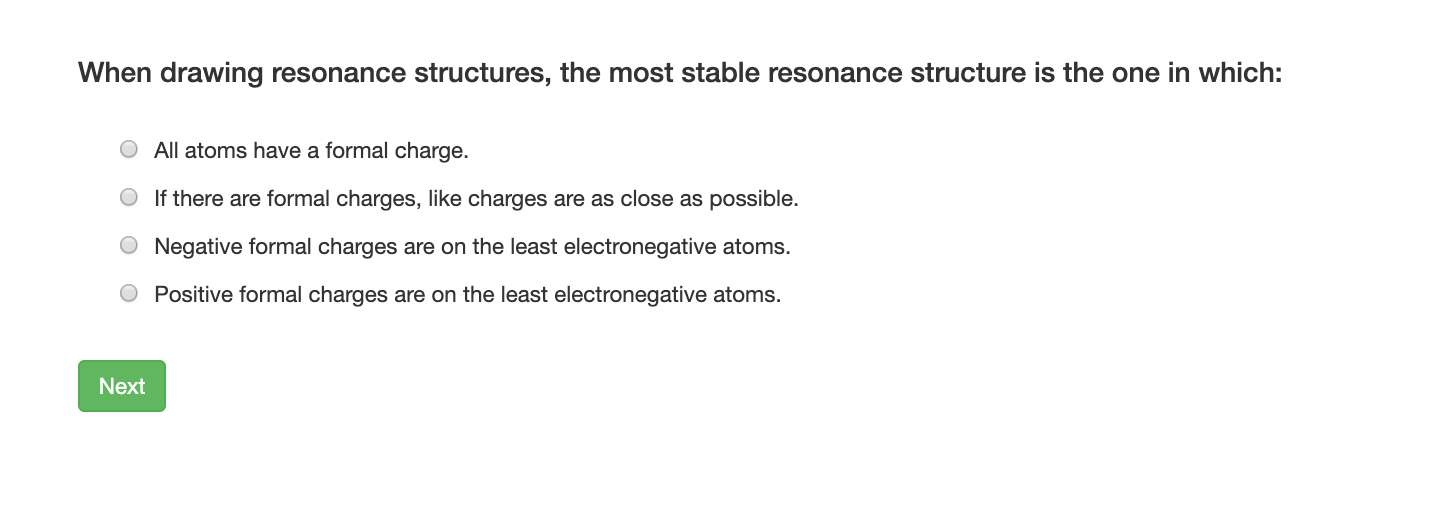WHATS THE ANSWER? When drawing resonance structures, the most stable resonance structure is the one in which: O All atoms have a formal charge. O If there are formal charges, like charges are as close as possible. O Negative formal charges are on the least electronegative atoms. O Positive formal charges are on the least electronegative atoms. Next

• ### Lewis structure help Number of Valence Electrons Molecule Number of Remaining Electrons Bond Skeleton Lewis...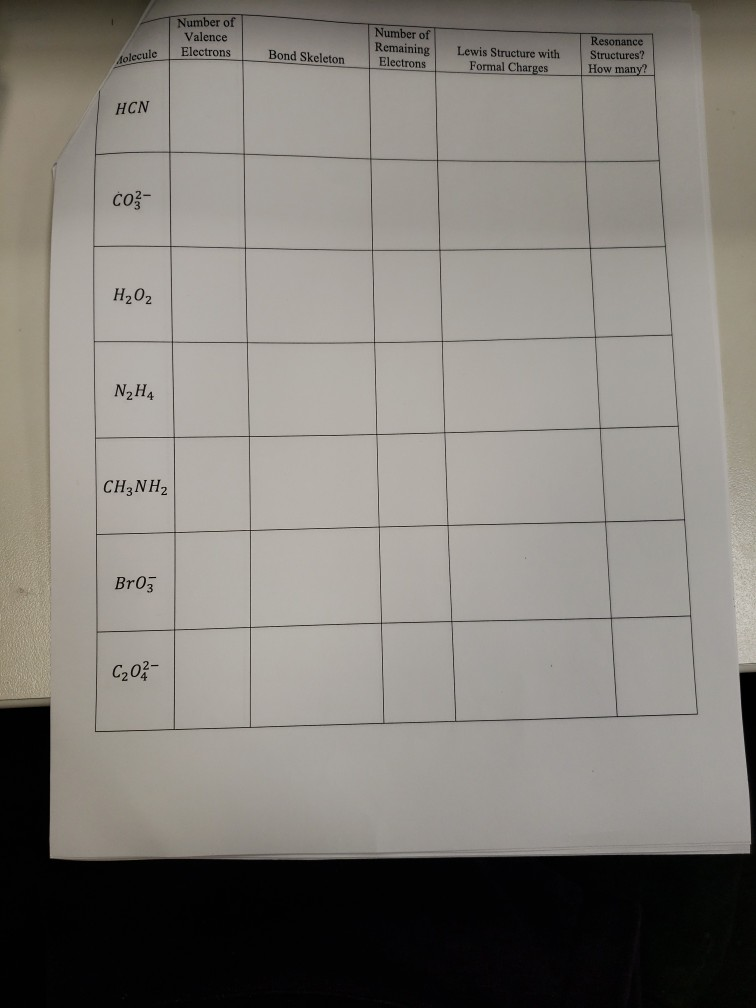Lewis structure help Number of Valence Electrons Molecule Number of Remaining Electrons Bond Skeleton Lewis Structure with Formal Charges Resonance Structures? How many? HCN coz- H₂O₂ N H4 CH3NH2 Br03 C202- Number of Valence Number of Remaining Electrons olecule Electrons Bond Skeleton Lewis Structure with Formal Charges Resonance Structures? How many? NO3 NO; HO H₃0* soz- CH,0 CH3OH or the molecule N, there are five possible Lewis Structures, Three have the N-N-O bond skeleton and wwo have the N-O-N bond...

• ### 2. (4 marks) A molecule with molecular formula CH2N2 has a C-N-N bond angle of 180°...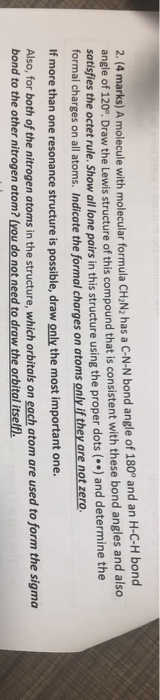2. (4 marks) A molecule with molecular formula CH2N2 has a C-N-N bond angle of 180° and an H-C-H bond angle of 120°. Draw the Lewis structure of this compound that is consistent with these bond angles and also satisfies the octet rule. Show all lone pairs in this structure using the proper dots (*) and determine the formal charges on all atoms. Indicate the formal charges on atoms only if they are not zero. If more than one resonance...

• ### Writing Lewis Structures d. Because oxygen is more electronegative than nitrogen, it is more likely to...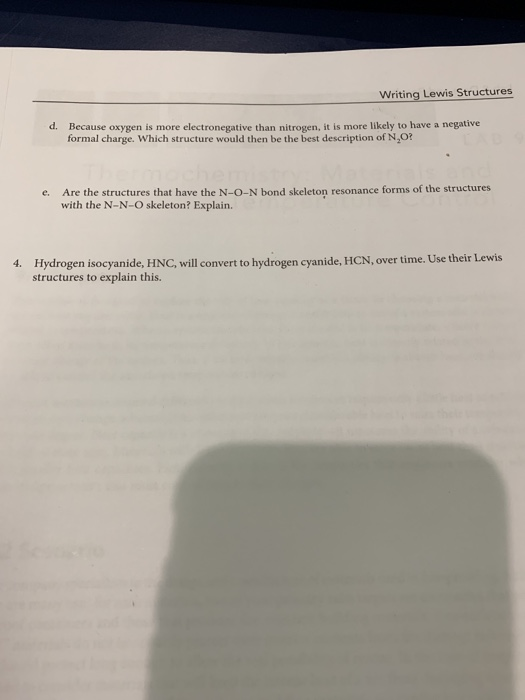Writing Lewis Structures d. Because oxygen is more electronegative than nitrogen, it is more likely to have a negative formal charge. Which structure would then be the best description of N,O? e. Are the structures that have the N-O-N bond skeleton resonance forms of the structures with the N-N-O skeleton? Explain. 4. Hydrogen isocyanide, HNC, will convert to hydrogen cyanide, HCN, over time. Use their Lewis structures to explain this.

• ### Consider the possible structures of the N ion shown below + N=N=N-NEN 2 :N-NEN-NEN NEN-N-NEN N-N=N=N=N...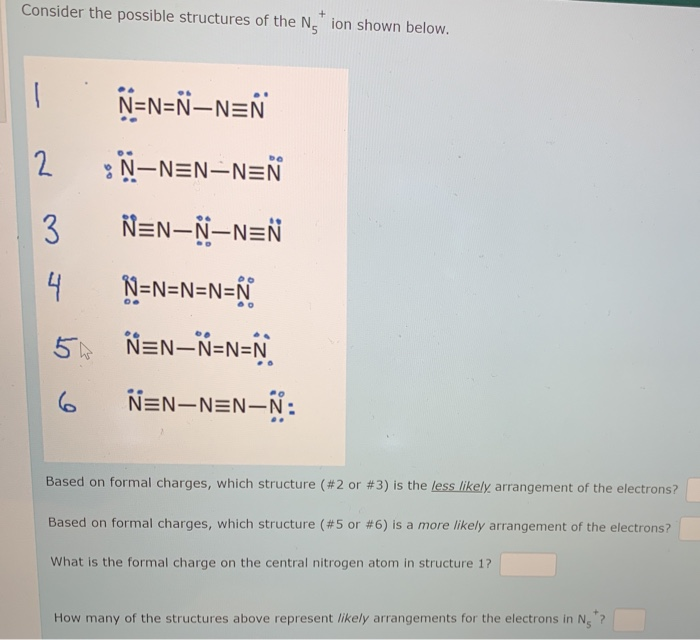Consider the possible structures of the N ion shown below + N=N=N-NEN 2 :N-NEN-NEN NEN-N-NEN N-N=N=N=N 5 NEN-N-N-N N=N-NEN-N: Based on formal charges, which structure ( #2 or #3) is the less likely arrangement of the electrons? Based on formal charges, which structure (#5 or #6 ) is a more likely arrangement of the electrons? What is the formal charge on the central nitrogen atom in structure 1? How many of the structures above represent likely arrangements for the electrons...

• ### #1 and #2 please. QUESTION 1 Check ALL that are true of formal charges. Formal charge...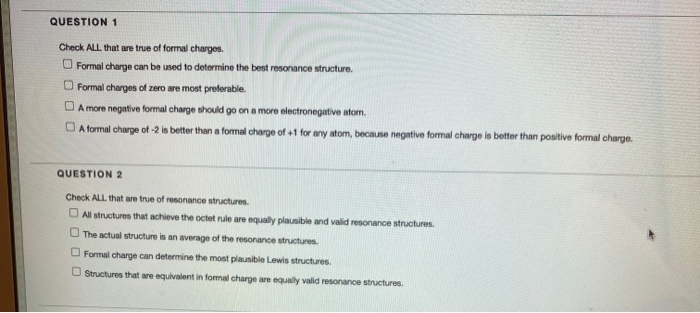#1 and #2 please. QUESTION 1 Check ALL that are true of formal charges. Formal charge can be used to determine the best resonance structure. Formal charges of zero are most preferable. A more negative formal charge should go on a more electronegative atom. A formal charge of -2 is better than a formal charge of +1 for any atom, because negative formal charge is better than positive formal charge. QUESTION 2 Check ALL that are true of resonance structures...

• ### Draw a Lewis structure for each of the following species. If more than one valid structure...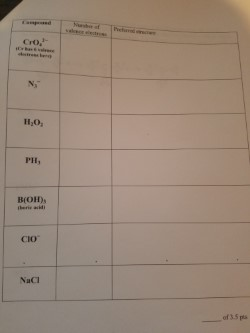Draw a Lewis structure for each of the following species. If more than one valid structure is possible, calculate formal charges to select the preferred structure. Be sure to include all lone pairs, radicals, and charges in the structure. Make sure the structure has the same number of electrons as the valence electrons in the compound. Cro 1H,O PH, B(OH)s CIo NaCl of 3.5 pa

• ### Which is not a stable molecule ? If you can explain me how to understand if...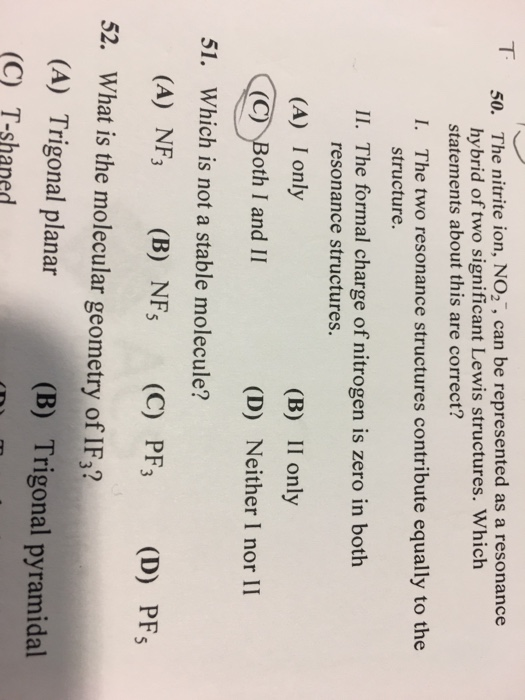Which is not a stable molecule ? If you can explain me how to understand if it is stable molecule or not , it will be appreciated 50. The nitrite ion, NO can be represented as a resonance hybrid of two significant Lewis structures. Which statements about this are correct? I. The two resonance structures contribute equally to the Structure. II. The formal charge of nitrogen is zero in both resonance structures. (A) I only (B) II only (C) oth...

• ### PRINTER VERSION « BACK Question 6 Pyridoxamine, aka vitamin B6, has the following structure. Which nitrogen...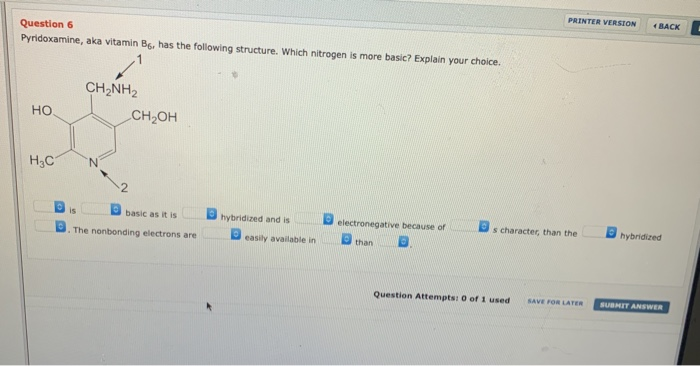PRINTER VERSION « BACK Question 6 Pyridoxamine, aka vitamin B6, has the following structure. Which nitrogen is more basic? Explain your choice. 1 CH_NH2 CH2OH HO H3C N 15 basic as it is The nonbonding electrons are hybridized and is easily available in electronegative because of than s character than the hybridized Question Attempts of 1 used SAVE FOR LATER SUBMIT ANSWER

• ### 22. Which of the following structure(s) contribute to the most stable NO2 structure? 10-N=0 II. I...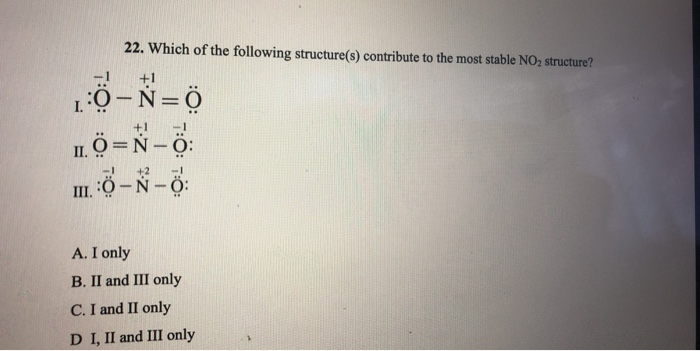22. Which of the following structure(s) contribute to the most stable NO2 structure? 10-N=0 II. I j=-ỏ: III. : -Ñ - A. I only B. II and III only C. I and II only D I, II and III only